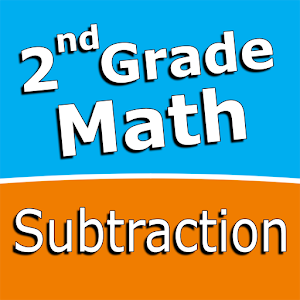Nederlands

We bieden dagelijks GRATIS gelicenseerde Android apps en games aan, die je normaal zou moeten kopen.\$2.99
VERSTREKEN

# Android Giveaway of the Day - Second grade Math - Subtraction

Intuitive interface powered by handwriting input and a fun and engaging mini game.
\$2.99 VERSTREKEN
Waarderingscijfer: 1 (100%) 0 (0%)

Deze giveaway is verlopen. Second grade Math - Subtraction is nu beschikbaar op de normale manier.

Intuitive interface powered by handwriting input and a fun and engaging mini game in addition to a regular math trainer mode make our app stand out from the crowd of generic math learning apps.

With Second grade Math - Subtraction you can practice and improve the following math skills:
- Subtraction facts - numbers up to 20
- Subtract a one-digit number from a two-digit number
- Subtract two two-digit numbers
- Subtract multiples of 10
- Subtract multiples of 100
- Subtract multiples of 10 or 100
- Subtract three-digit numbers
- Balance subtraction equations

Sergey Malugin

Education

### Versie:

Varies with device

### Grootte:

Varies with device

Everyone

### Compatibiliteit:

Varies with device

## Reactie op Second grade Math - Subtraction

Thank you for voting!